« Vrati se

#### MEMO 2012 ekipno problem 4

Let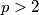$p>2$ be a prime number. For any permutation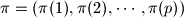$\pi = ( \pi(1) , \pi(2) , \cdots , \pi(p) )$ of the set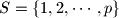$S = \{ 1, 2, \cdots , p \}$, let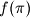$f( \pi )$ denote the number of multiples of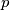$p$ among the following$p$ numbers: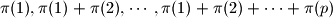Determine the average value of$f( \pi)$ taken over all permutations$\pi$ of$S$.